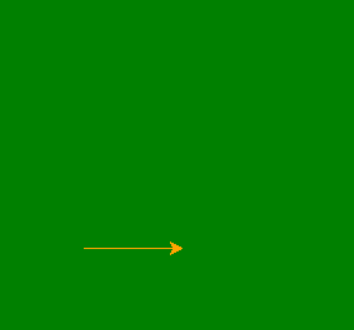# HSSlive: Plus One & Plus Two Notes & Solutions for Kerala State Board

## How to Draw a Cube Using Python Turtle, Tkinter, Matplotlib & Without Libraries

How to Draw a Cube in Python - There are various methods to create a Cube using python libraries like turtle, tkinter and matplotlib. Also, you will learn how to make a Cube using python in easy and simple steps. We have also provided the python code for drawing a Cube pattern. If you have introductory to intermediate knowledge in Python and statistics, then you can use this article as a one-stop shop for building and plotting Cube patterns in Python using libraries from its scientific stack, including NumPy, Matplotlib, Pandas, and Seaborn.

Plotting a Cube in python is a simple procedure, and python in its simplest provides multiple easy methods to do so.

## How to Draw a Cube using Python?

Given below is the process to draw a Cube in python using libraries like Turtle, Tkinter, Matplotib and others.

Four Method to make a Cube in Python using multiple libraries:

1. How to draw a Cube using python turtle?
2. How to draw a Cube using python tkinter?
3. How to draw a Cube using python matplotlib?
4. How to draw a Cube using python code without library?

## How to Draw a Cube using Python Turtle?

Check below step by step process to make a Cube using the python turtle library.

Step

#### Drawing Cube

Following steps are used:

• First draw the front square
• Move to back square through one bottom left side
• Draw the back square
• Draw the remaining side as shown in code

Code

#import the turtle modules
import turtle

# Forming the window screen
tut = turtle.Screen()

# background color green
tut.bgcolor("green")

# window title Turtle
tut.title("Turtle")
my_pen = turtle.Turtle()

# object color
my_pen.color("orange")
tut = turtle.Screen()

# forming front square face
for i in range(4):
my_pen.forward(100)
my_pen.left(90)

# bottom left side
my_pen.goto(50,50)

# forming back square face
for i in range(4):
my_pen.forward(100)
my_pen.left(90)

# bottom right side
my_pen.goto(150,50)
my_pen.goto(100,0)

# top right side
my_pen.goto(100,100)
my_pen.goto(150,150)

# top left side
my_pen.goto(50,150)
my_pen.goto(0,100)

OutputHow to Draw a Cube Using Python Turtle, Tkinter, Matplotlib & Without Libraries

## How to Draw a Cube using Python Tkinter?

Check below step by step process to make a Cube using the python tkinter library.

Step

Code

Output

## How to Draw a Cube using Python Matplotlib?

Check below step by step process to make a Cube using the python matplotlib library.

Step

Code

Output

## How to Draw a Cube using Python Code without Library?

Step

Code

Output

Note: Also, these codes might not run in an online compiler please use an offline compiler. Programmers can make any changes in the code according to their specific requirement.

## Conclusion on How to Draw a Cube using Python

We hope the programs and methods provided above on how to make a Cube using python libraries have been helpful to you. If there is any issue in any of the code please let us know in the comments. We will try to resolve it as soon as possible.

Share: